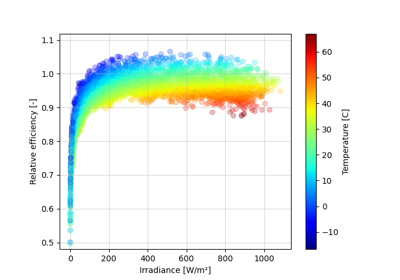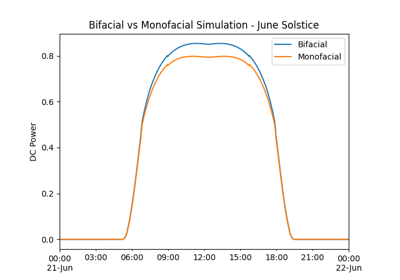# pvlib.temperature.faiman#

pvlib.temperature.faiman(poa_global, temp_air, wind_speed=1.0, u0=25.0, u1=6.84)[source]#

Calculate cell or module temperature using the Faiman model.

The Faiman model uses an empirical heat loss factor model 1 and is adopted in the IEC 61853 standards 2 and 3.

Usage of this model in the IEC 61853 standard does not distinguish between cell and module temperature.

Parameters
• poa_global (numeric) – Total incident irradiance [W/m^2].

• temp_air (numeric) – Ambient dry bulb temperature [C].

• wind_speed (numeric, default 1.0) – Wind speed in m/s measured at the same height for which the wind loss factor was determined. The default value 1.0 m/s is the wind speed at module height used to determine NOCT. [m/s]

• u0 (numeric, default 25.0) – Combined heat loss factor coefficient. The default value is one determined by Faiman for 7 silicon modules in the Negev desert on an open rack at 30.9° tilt. $$\left[\frac{\text{W}/{\text{m}^2}}{\text{C}}\right]$$

• u1 (numeric, default 6.84) – Combined heat loss factor influenced by wind. The default value is one determined by Faiman for 7 silicon modules in the Negev desert on an open rack at 30.9° tilt. $$\left[ \frac{\text{W}/\text{m}^2}{\text{C}\ \left( \text{m/s} \right)} \right]$$

Returns

numeric, values in degrees Celsius

Notes

All arguments may be scalars or vectors. If multiple arguments are vectors they must be the same length.

References

1

Faiman, D. (2008). “Assessing the outdoor operating temperature of photovoltaic modules.” Progress in Photovoltaics 16(4): 307-315. DOI: 10.1002/pip.813

2

“IEC 61853-2 Photovoltaic (PV) module performance testing and energy rating - Part 2: Spectral responsivity, incidence angle and module operating temperature measurements”. IEC, Geneva, 2018.

3

“IEC 61853-3 Photovoltaic (PV) module performance testing and energy rating - Part 3: Energy rating of PV modules”. IEC, Geneva, 2018.

## Examples using pvlib.temperature.faiman#Simulating PV system DC output using the ADR module efficiency model

Simulating PV system DC output using the ADR module efficiency modelBifacial Modeling - procedural

Bifacial Modeling - procedural